### Home > CCG > Chapter 2 > Lesson 2.1.1 > Problem2-9

2-9.

Mei puts the shapes below into a bucket and asks Brian to pick one out.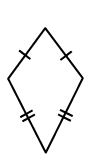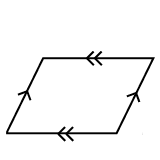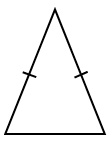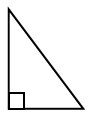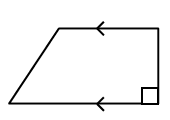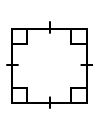1. What is the probability that he pulls out a quadrilateral with parallel sides?

Identify the shapes that are quadrilaterals with a $Q$.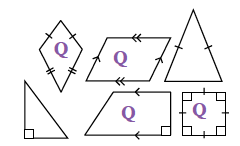Identify the shapes that have parallel sides with a $P$.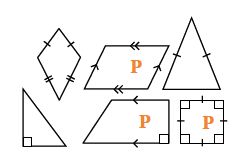Write a fraction where the numerator is the number of shapes that have both parallel sides and four sides, and where the denominators the total number of shapes.

$\frac{3}{6}=\frac{1}{2}$

2. What is the probability that he pulls out a shape with rotation symmetry?